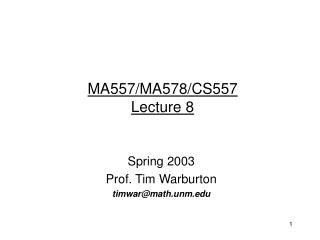Download PresentationMA557/MA578/CS557 Lecture 8

# MA557/MA578/CS557 Lecture 8 - PowerPoint PPT PresentationDownload Presentation## MA557/MA578/CS557 Lecture 8

- - - - - - - - - - - - - - - - - - - - - - - - - - - E N D - - - - - - - - - - - - - - - - - - - - - - - - - - -
##### Presentation Transcript

1. MA557/MA578/CS557Lecture 8 Spring 2003 Prof. Tim Warburton timwar@math.unm.edu

2. Discontinuous Galerkin Scheme • Recall that under sufficient conditions (i.e. smoothness) on an interval we obtained the density advection PDE • We again divide the pipe into sections divided by x1,x2,…,xN . • On each section we are going to solve a weak version of the advection equation.

3. x1 x2 x3 xN xN-1 In the i’th cell we will calculate an approximation of q, denoted by . Typically, this will be a p’th order polynomial on the i’th interval. We will solve the following weak equation for i=1,..,N-1

4. Discretization of Pp • In order to work with the DG scheme we need to choose a basis for Pp and formulate the scheme as a finite dimensional problem • We expand the density in terms of the Legendre polynomials

5. Discretization of Pp • The scheme: • Becomes:

6. Simplify, simplify • Starting with: • We define two matrices:

7. Then: Becomes:

8. Questions • This raises the following questions how can we compute Mnm and Dnm • We will need some of the following results.

9. The Legendre Polynomials(used to discretize Pp) • Let’s introduce the Legendre polynomials. • The Legendre polynomials are defined as eigenfunctions of the singular Sturm-Liouville problem:

10. Closed Form Formula for Lm • If we choose Lm(1) =1 then: • Examples: • Warning: this is not a stable way to compute the value of a Legendre polynomial (unstable as m increases)

11. Stable Formula for Computing Lm • We can also use the following recurrence relation to compute Lm • Starting with L0=1 L1=x we obtain: • This is a more stable way to compute the Legendre polynomials at some x.

12. The Rodriguez Formula • We can also obtain the m’th order Legendre polynomial using the Rodriguez formula:

13. Orthogonality of the Legendre Polynomials • The Legendre polynomials are orthogonal to each other: • Since 2m < m+p

14. Miscellaneous Relations

15. Differentiation • Suppose we have a Legendre expansion for the density in a cell: • We can find the coefficients of the Legendre expansion for the derivative of the function as:where:

16. Coefficient Differentiation Matrix i.e. if we create a vector of the Legendre coefficients for rho in the I’th cell then we can compute the Legendre coefficients of the derivative of rho in the I’th cell by pre-multiplying the vector of coefficients with the matrix:

17. The D Matrix • We can use a simple trick to compute D • Recall: • We use:

18. The Vandermonde Matrix • It will be convenient for future applications to be able to transform between data at a set of nodes and the Legendre coefficients of the p’th order polynomial which interpolates the data at these nodes. • Consider the I’th cell again, but imagine that there are p+1 nodes in that cell then we have: • Notice that the Vandermonde matrix defined by is square and we can obtain the coefficients by multiplying both sides by the inverse of V

19. Vandermonde • Reiterate: • that the inverse of the Vandermonde matrix is very useful for transforming nodal data to Legendre coefficients • that the Vandermonde matrix is very useful for transforming Legendre coefficients to nodal data

20. Results So Far • 0<=n,j,m<=p Mass matrix: Coeff. Differentiation Stiffness matrix Vandermonde matrix

21. Homework 3 Q1) Code up a function (LEGENDRE) which takes a vector x and m as arguments and computes the m’th order Legendre polynomial at x using the stable recurrence relation. Q2) Create a function (LEGdiff) which takes p as argument and returns the coefficient differentiation matrix D Q3) Create a function (LEGmass) which takes p as argument and returns the mass matrix M

22. Homework 3 cont Q4) Create a function LEGvdm which returns the following matrix: Q5) Test them with the following matlab routine: • Compute the Chebychev nodes • Build the Vandermonde matrix using theChebychev nodes and Legendre polynomials • Build the coefficient differentiation matrix • 9) Build the Legendre mass matrix • 12) Evaluate x^m at the Chebychev nodes • 14) Compute the Legendre coefficients • 16) Multiply by Dhat (i.e. differentiate in coeff space) • 18) Evaluate at the nodes • 20) Compute the error in the derivative

23. Homework 3 cont Q5cont) Explain the output from this test case

24. Next Lecture (9) • We will put everything together to build a 1D DG scheme for advection…